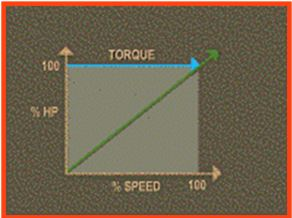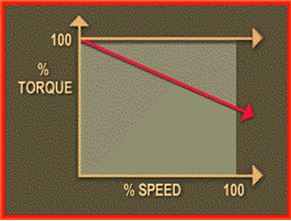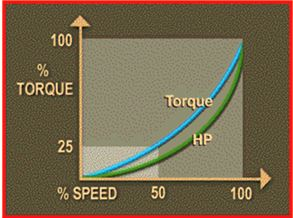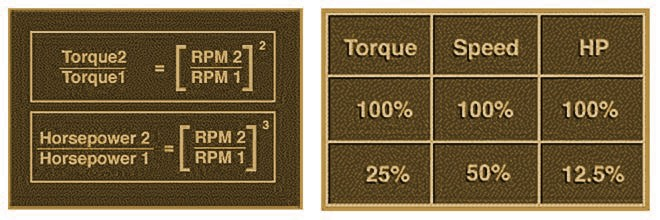# 3 Types of Loads (Constant Torque/Constant Power/Variable Torque)

#### Type 1 – CONSTANT TORQUE LOADSConstant torque loads require the same amount of torque at low speeds as at high speeds. Torque remains constant throughout the speed range, and the horsepower increases and decreases in direct proportion to the speed. Constant torque loads include most positive displacement and reciprocating pumps and compressors as well as traction drives and conveyors. With constant torque loads, the torque is not a function of speed. As speed is changed, the load torque will remain fairly constant and the horsepower will change linearly with the speed.

For example, if the speed increases by 50%, then the power required to drive the operation will increase 50% while the torque remains constant.

#### Type 2 – CONSTANT POWER LOADSConstant horsepower loads require high torque at low speeds and low torque at high speeds, which means constant horsepower at any speed. Constant horsepower loads include grinders, winding machines and lathes. For constant horsepower loads, the torque loading is a function of speed up to 100% operating speed. As the speed of the operation is decreased, the torque increases so that the horsepower required remains essentially constant.

#### Type 3 – VARIABLE TORQUE LOADSVariable torque loads require much lower torque at low speeds than at high speeds. The torque required varies as the square of the speed and the horsepower required varies as the cube of the speed. Variable torque loads include most centrifugal and axial pumps, fans and blowers and many mixers and agitators.

As the speed is decreased, the torque will decrease by the square of the speed decrease and the horsepower required decreases by the cube of the speed decrease.As an example, when the speed of a variable torque load is reduced by 50% or one half, the torque required to drive the load is reduced to one-quarter or 25%. The horsepower is reduced to the speed cubed, which is 1/8, or 12.5% of that required to drive the load at full speed.